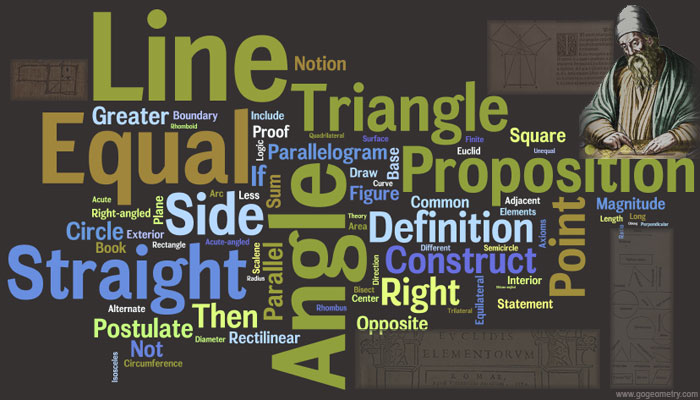Euclid's Elements Book I Word CloudEuclid's Elements Euclid's Elements is a mathematical and geometric treatise consisting of 13 books written by the Greek mathematician Euclid in Alexandria circa 300 BC. It comprises a collection of definitions, postulates (axioms), propositions (theorems and constructions), and mathematical proofs of the propositions. Book 1 contains Euclid's 10 axioms (5 named postulates - including the parallel postulate - and 5 named axioms) and the basic propositions of geometry: the pons asinorum (proposition 5) , the Pythagorean theorem (Proposition 47), equality of angles and areas, parallelism, the sum of the angles in a triangle, and the three cases in which triangles are "equal" (have the same area). Word Cloud Word cloud or tag cloud (or weighted list in visual design) is a visual depiction of user-generated tags, or simply the word content of a site, used typically to describe the content of web sites. Tags are usually single words and are typically listed alphabetically, and the importance of a tag is shown with font size or color. Thus both finding a tag by alphabet and by popularity is possible. The tags are usually hyperlinks that lead to a collection of items that are associated with a tag. The word cloud image was generated by Wordle.net. Recent Additions